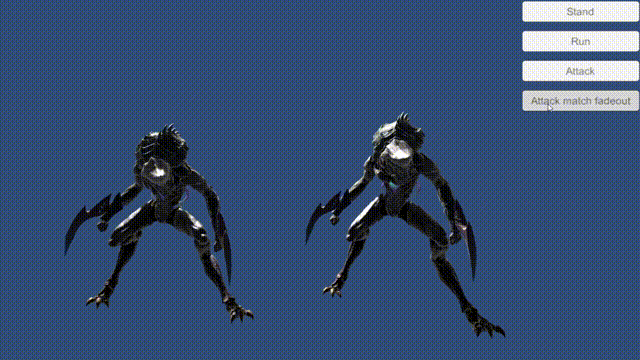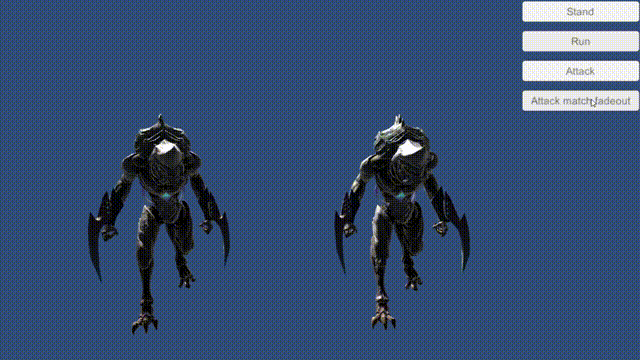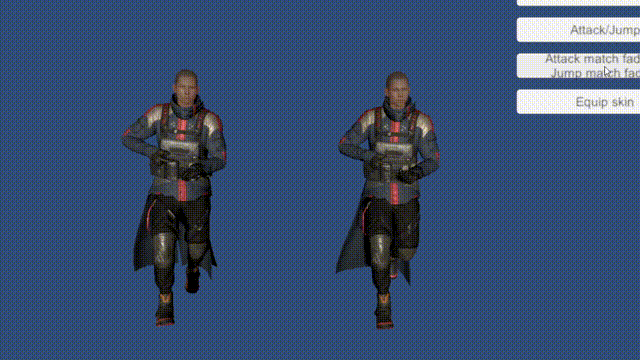# LPQ-Blog

Learn something...

liu_peiqi@163.com

## 姿态相似

$\begin{Vmatrix} \ & pos & rot & scale \\ root & \ddots \\ bip1 \ & \ & \ddots \\ bip2 & \ & \ & \ddots \end{Vmatrix}$

$Cos(Mi,Mj) = \sum \frac {Mi_n \cdot Mj_n} {\left\| Mi_n \right\| \times \left\| Mj_n \right\|} \div n$

## 动作融合的相似矩阵

$clip: attack \begin{Vmatrix} \ & stand & walk & run\\ t0 & (t0:0.99) & (t26:0.93) & (t13:0.59)\\ t1 & (t49:0.79) & (t29:0.86) & (t9:0.60)\\ t2 & (t50:0.74) & (t30:0.83) & (t9:0.59)\\ \dots & \dots & \dots & \dots \\ t37 & (t7:0.97) & (t29:0.96) & (t12:0.61)\\ t38 & (t4:0.99) & (t27:0.95) & (t12:0.60)\\ \end{Vmatrix}$## 筛选最佳衔接动作

$clip:run \begin{Vmatrix} \ & stand\ jump & left\ jump & right\ jump \\ t0 & (t0:0.89) & (t0:0.92) & (t0:0.94) \\ t1 & (t0:0.89) & (t0:0.91) & (t0:0.93) \\ t2 & (t0:0.88) & (t0:0.90) & (t0:0.92) \\ \dots & \dots & \dots & \dots \\ t16 & (t0:0.90) & (t0:0.93) & (t0:0.94) \\ t17 & (t0:0.90) & (t0:0.93) & (t0:0.94) \\ \end{Vmatrix}$

## 把运动趋势考虑进来

$Cos(Mi_{[m,m+n)},Mj_{[0,n)}) = \frac { \sum_{k=0}^n{Cos(Mi_{m+k}, Mj_k)}} {n}$

1. 当前动作执行到了run动作的第t10帧，执行起跳命令
2. 在衔接相似矩阵里连续筛选了5行数据，比如t10-t14
3. 找到相似值最大的元素
4. 假设位于t13行，left jump列。
5. 得到的最佳帧和当前帧还差3帧，那么就延迟3帧播放left jump动作。